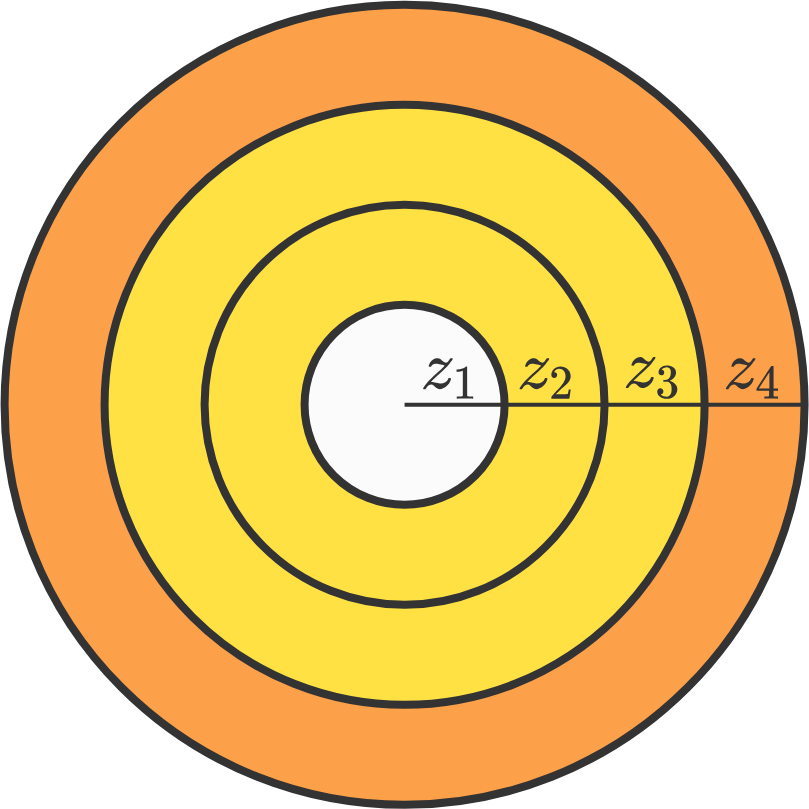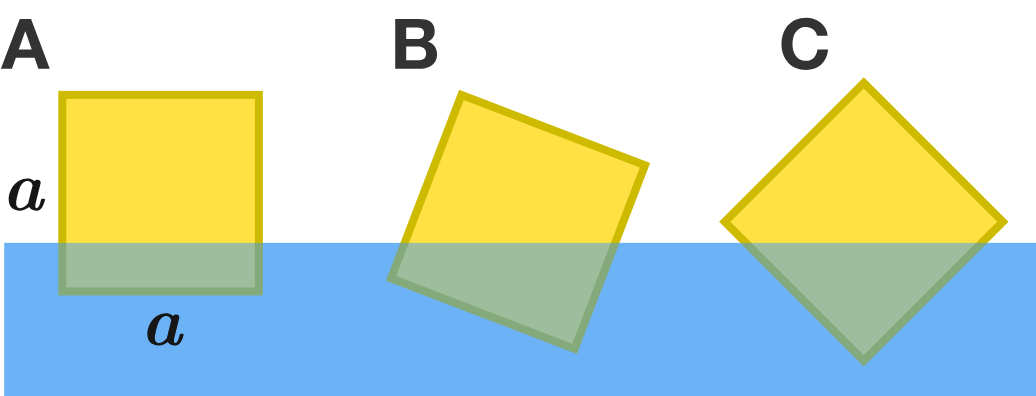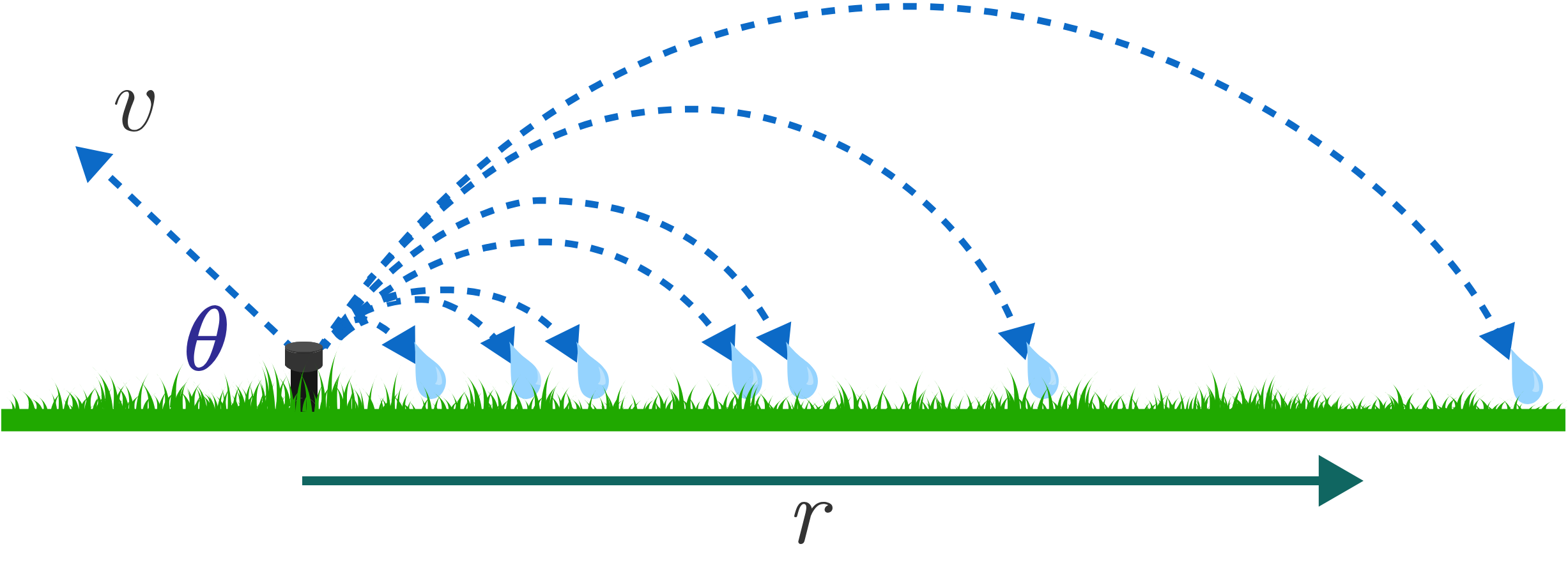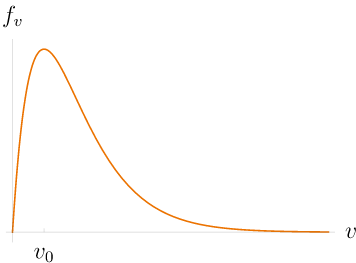# Problems of the Week

Contribute a problem

In this diagram of four concentric circles, the combined area of the yellow regions is the same as the combined area of the orange and white regions.

$z_1, z_2, z_3,$ and $z_4$ are positive integers such that $\gcd (z_1,z_2,z_3,z_4) = 1$.

Must it be true that $z_1 = z_2 = z_3 = z_4?$Diagram not necessarily drawn to scale.

If $x$ is chosen uniformly at random on the interval $(0,1],$ then what is the probability that $\left\lfloor \frac{\lfloor x\rfloor+1}{x} \right\rfloor \leq 3?$

Notation: $\lfloor \cdot \rfloor$ denotes the floor function.

Write down all the integers 1 to 1,000,000 in a row.

• Cross out all of the odd numbers.
• Of the remaining numbers, cross out all of those that are now in even positions (i.e. cross out 4, 8, 12, 16, ...).
• Of the remaining numbers, cross out all of those that are now in odd positions.
• Continue crossing out numbers in this manner, alternating at each stage between even and odd positions, until one number remains.

Let $A$ be this last number standing.

Now, write down the numbers 1 to one million again. Repeat the process, but this time with the words "even" and "odd" switched. Let $B$ be the last number remaining in this case.

What is the sum $A+B?$A cuboid block of dimensions $a \times a \times c$ $($with $a\ll c)$ and uniform density $\rho<0.5$ floats on water. Depending on the density $\rho$, there are three different equilibrium possibilities, illustrated in the figure.

A. The bottom side is horizontal.

B. The bottom side makes an angle of $\alpha<45^{\circ}$ to the horizontal. Note that there are two possibilities, one with a leftward tilt and the other with a rightward tilt, and these two configurations are equally stable.

C. The block floats so that two bottom sides make $45^{\circ}$ to the horizontal.

For densities close to $\rho=0.5,$ C correctly describes the stable equilibrium. Configurations B and A follow as the density decreases and approaches $\rho=0.0$.

What is the minimum density for configuration C to be stable?

Notes: The density $\rho$ is measured relative to the water. For $\rho>0.5,$ the stable configurations follow in reverse order, with configuration A being stable for $\rho$ approaching 1, except for the fact that the block is much more submerged.A stationary lawn sprinkler waters a circular region of grass by launching water droplets at an angle of $\theta=30^\circ$ above the horizontal in all directions.The water droplets leave the sprinkler with a range of speeds $0 \lt v \lt \infty$ according to the velocity distribution $f_v(v)=\frac{v}{\ v_0^2\ }e^{-v/v_0}.$ The probability that a droplet is launched with a speed between $v$ and $v+dv$ is $f_v(v) dv,$ so the most likely speed is $v_0$, the maximum of $f_v.$

If the droplets follow parabolic trajectories with acceleration $g$ toward the ground, find the probability distribution describing the distance $r$ where a droplet lands on the grass.

What is the most likely distance (in meters) from the sprinkler that a droplet lands?

Assumptions:

• Air drag is negligible.
• The height from which the droplets are launched is negligible.
• The most likely speed of a droplet is $v_0=\SI[per-mode=symbol]{1}{\meter\per\second},$ and $g=\SI[per-mode=symbol]{10}{\meter\per\second\squared}.$
×